## Electrostatics Exam1 and Problem Solutions

Electrostatics Exam1 and  Problem Solutions

1. If we touch two spheres to each other, find the final charges of the spheres.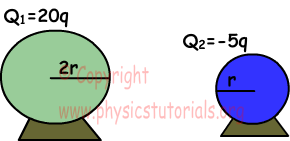Charge per unit radius is found;

qr=(Q1+Q2)/(r1+r2)

qr=(20-5)q/(2r+r)=5q/r

Charge of first sphere becomes;

Q1=qr.r1=5q/r.2r=10q

Charge of second sphere becomes;

Q2=qr.r2=5q/r.r=5q

2. Positively charged sphere B is placed between two neutral spheres A and C. We cut connection of A and C with ground. If we put A closer to the first electroscope and touch C to the sphere of second electroscope, find the type of charge electroscopes have.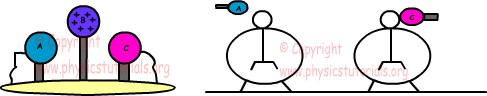A and C are negatively charged by induction. Thus, leaves of both electroscopes are negatively charged.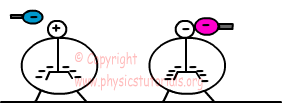3. If force applied by charge placed at point B on A is F, find forces applied by charges C and D on A in terms of F.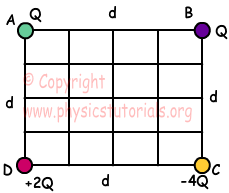Free body diagram of forces is given below;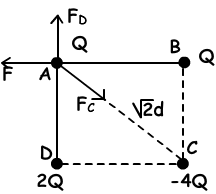F=k.Q.Q/d2=k.Q2/d2

FC=k.Q.(-4Q)/(√2d)2=-4k.Q2/2d2=-2.k.Q2/d2=-2F

FD=k.Q.2Q/d2=2k.Q2/d2=2F

4. Find the electric field at point A produced by charges q1 and q2 in terms of k, q and d.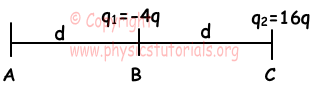We assume that there is a +q charge at point A while finding electric field at point A.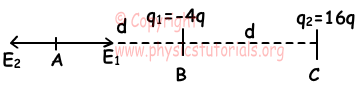E1=k.(-4q)/d2=-4k.q/d2

E2=k.(16q)/4d2=4k.q/d2

Resultant electric field at point A is;

Eresultant=E1+E2=-4kq/d2+4kq/d2=0

5. Find electric potential energy produced by Q1, Q2 and Q3 in terms of k.q2/r.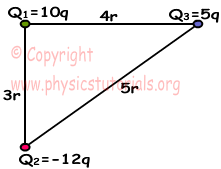Ep=k.Q1.Q2/r

EP1,2=k.10q.(-12q)/3r=-40k.q2/r

EP1,3=k.10q.5q/4r=25k.q2/2r

EP2,3=k.(-12q).5q/5r=-12k.q2/r

EP=EP1,2+EP1,3+EP2,3

EP=-40k.q2/r+25k.q2/2r-12k.q2/r

EP=-39,5k.q2/r

Author: